In :
!unzip "drive/My Drive/Applied_AI/HAR/HumanActivityRecognition.zip"

In :
from google.colab import drive
drive.mount('/content/drive')

Go to this URL in a browser: https://accounts.google.com/o/oauth2/auth?client_id=947318989803-6bn6qk8qdgf4n4g3pfee6491hc0brc4i.apps.googleusercontent.com&redirect_uri=urn%3Aietf%3Awg%3Aoauth%3A2.0%3Aoob&scope=email%20https%3A%2F%2Fwww.googleapis.com%2Fauth%2Fdocs.test%20https%3A%2F%2Fwww.googleapis.com%2Fauth%2Fdrive%20https%3A%2F%2Fwww.googleapis.com%2Fauth%2Fdrive.photos.readonly%20https%3A%2F%2Fwww.googleapis.com%2Fauth%2Fpeopleapi.readonly&response_type=code

··········
Mounted at /content/drive


# HumanActivityRecognition¶

This project is to build a model that predicts the human activities such as Walking, Walking_Upstairs, Walking_Downstairs, Sitting, Standing or Laying.

This dataset is collected from 30 persons(referred as subjects in this dataset), performing different activities with a smartphone to their waists. The data is recorded with the help of sensors (accelerometer and Gyroscope) in that smartphone. This experiment was video recorded to label the data manually.

## How data was recorded¶

By using the sensors(Gyroscope and accelerometer) in a smartphone, they have captured '3-axial linear acceleration'(tAcc-XYZ) from accelerometer and '3-axial angular velocity' (tGyro-XYZ) from Gyroscope with several variations.

prefix 't' in those metrics denotes time.

suffix 'XYZ' represents 3-axial signals in X , Y, and Z directions.

### Feature names¶

1. These sensor signals are preprocessed by applying noise filters and then sampled in fixed-width windows(sliding windows) of 2.56 seconds each with 50% overlap. ie., each window has 128 readings.

2. From Each window, a feature vector was obtianed by calculating variables from the time and frequency domain.

In our dataset, each datapoint represents a window with different readings

3. The accelertion signal was saperated into Body and Gravity acceleration signals(tBodyAcc-XYZ and tGravityAcc-XYZ) using some low pass filter with corner frequecy of 0.3Hz.

4. After that, the body linear acceleration and angular velocity were derived in time to obtian jerk signals (tBodyAccJerk-XYZ and tBodyGyroJerk-XYZ).

5. The magnitude of these 3-dimensional signals were calculated using the Euclidian norm. This magnitudes are represented as features with names like tBodyAccMag, tGravityAccMag, tBodyAccJerkMag, tBodyGyroMag and tBodyGyroJerkMag.

6. Finally, We've got frequency domain signals from some of the available signals by applying a FFT (Fast Fourier Transform). These signals obtained were labeled with prefix 'f' just like original signals with prefix 't'. These signals are labeled as fBodyAcc-XYZ, fBodyGyroMag etc.,.

7. These are the signals that we got so far.

• tBodyAcc-XYZ
• tGravityAcc-XYZ
• tBodyAccJerk-XYZ
• tBodyGyro-XYZ
• tBodyGyroJerk-XYZ
• tBodyAccMag
• tGravityAccMag
• tBodyAccJerkMag
• tBodyGyroMag
• tBodyGyroJerkMag
• fBodyAcc-XYZ
• fBodyAccJerk-XYZ
• fBodyGyro-XYZ
• fBodyAccMag
• fBodyAccJerkMag
• fBodyGyroMag
• fBodyGyroJerkMag
8. We can esitmate some set of variables from the above signals. ie., We will estimate the following properties on each and every signal that we recoreded so far.

• mean(): Mean value
• std(): Standard deviation
• max(): Largest value in array
• min(): Smallest value in array
• sma(): Signal magnitude area
• energy(): Energy measure. Sum of the squares divided by the number of values.
• iqr(): Interquartile range
• entropy(): Signal entropy
• arCoeff(): Autorregresion coefficients with Burg order equal to 4
• correlation(): correlation coefficient between two signals
• maxInds(): index of the frequency component with largest magnitude
• meanFreq(): Weighted average of the frequency components to obtain a mean frequency
• skewness(): skewness of the frequency domain signal
• kurtosis(): kurtosis of the frequency domain signal
• bandsEnergy(): Energy of a frequency interval within the 64 bins of the FFT of each window.
• angle(): Angle between to vectors.
9. We can obtain some other vectors by taking the average of signals in a single window sample. These are used on the angle() variable' 

• gravityMean
• tBodyAccMean
• tBodyAccJerkMean
• tBodyGyroMean
• tBodyGyroJerkMean

### Y_Labels(Encoded)¶

• In the dataset, Y_labels are represented as numbers from 1 to 6 as their identifiers.

• WALKING as 1
• WALKING_UPSTAIRS as 2
• WALKING_DOWNSTAIRS as 3
• SITTING as 4
• STANDING as 5
• LAYING as 6

## Train and test data were saperated¶

• The readings from 70% of the volunteers were taken as trianing data and remaining 30% subjects recordings were taken for test data

## Data¶

• All the data is present in 'UCI_HAR_dataset/' folder in present working directory.
• Feature names are present in 'UCI_HAR_dataset/features.txt'
• Train Data
• 'UCI_HAR_dataset/train/X_train.txt'
• 'UCI_HAR_dataset/train/subject_train.txt'
• 'UCI_HAR_dataset/train/y_train.txt'
• Test Data
• 'UCI_HAR_dataset/test/X_test.txt'
• 'UCI_HAR_dataset/test/subject_test.txt'
• 'UCI_HAR_dataset/test/y_test.txt'

27 MB

# Quick overview of the dataset :¶

• Accelerometer and Gyroscope readings are taken from 30 volunteers(referred as subjects) while performing the following 6 Activities.

1. Walking
2. WalkingUpstairs
3. WalkingDownstairs
4. Standing
5. Sitting
6. Lying.
• Readings are divided into a window of 2.56 seconds with 50% overlapping.

• Accelerometer readings are divided into gravity acceleration and body acceleration readings, which has x,y and z components each.

• Gyroscope readings are the measure of angular velocities which has x,y and z components.

• Jerk signals are calculated for BodyAcceleration readings.

• Now, on all the base signal readings., mean, max, mad, sma, arcoefficient, engerybands,entropy etc., are calculated for each window.

• We get a feature vector of 561 features and these features are given in the dataset.

• Each window of readings is a datapoint of 561 features.

## Problem Framework¶

• 30 subjects(volunteers) data is randomly split to 70%(21) test and 30%(7) train data.
• Each datapoint corresponds one of the 6 Activities.

## Problem Statement¶

• Given a new datapoint we have to predict the Activity
In :
import numpy as np
import pandas as pd

# get the features from the file features.txt
features = list()
with open('drive/My Drive/Applied_AI/HAR/UCI_HAR_Dataset/features.txt') as f:
features = [line.split() for line in f.readlines()]
print('No of Features: {}'.format(len(features)))

No of Features: 561

In :
%matplotlib inline
import matplotlib.pyplot as plt
import numpy as np
import time
# https://gist.github.com/greydanus/f6eee59eaf1d90fcb3b534a25362cea4
# https://stackoverflow.com/a/14434334
# this function is used to update the plots for each epoch and error
def plt_dynamic(x, vy, ty, ax, colors=['b']):
ax.plot(x, vy, 'b', label="Validation Loss")
ax.plot(x, ty, 'r', label="Train Loss")
plt.legend()
plt.grid()
fig.canvas.draw()


## Obtain the train data¶

In :
# get the data from txt files to pandas dataffame

# add subject column to the dataframe

y_train = pd.read_csv('drive/My Drive/Applied_AI/HAR/UCI_HAR_Dataset/train/y_train.txt', names=['Activity'], squeeze=True)
y_train_labels = y_train.map({1: 'WALKING', 2:'WALKING_UPSTAIRS',3:'WALKING_DOWNSTAIRS',\
4:'SITTING', 5:'STANDING',6:'LAYING'})

# put all columns in a single dataframe
train = X_train
train['Activity'] = y_train
train['ActivityName'] = y_train_labels
train.sample()

/usr/local/lib/python3.6/dist-packages/pandas/io/parsers.py:702: UserWarning: Duplicate names specified. This will raise an error in the future.

Out:
tBodyAcc-mean()-X tBodyAcc-mean()-Y tBodyAcc-mean()-Z tBodyAcc-std()-X tBodyAcc-std()-Y tBodyAcc-std()-Z tBodyAcc-mad()-X tBodyAcc-mad()-Y tBodyAcc-mad()-Z tBodyAcc-max()-X tBodyAcc-max()-Y tBodyAcc-max()-Z tBodyAcc-min()-X tBodyAcc-min()-Y tBodyAcc-min()-Z tBodyAcc-sma() tBodyAcc-energy()-X tBodyAcc-energy()-Y tBodyAcc-energy()-Z tBodyAcc-iqr()-X tBodyAcc-iqr()-Y tBodyAcc-iqr()-Z tBodyAcc-entropy()-X tBodyAcc-entropy()-Y tBodyAcc-entropy()-Z tBodyAcc-arCoeff()-X,1 tBodyAcc-arCoeff()-X,2 tBodyAcc-arCoeff()-X,3 tBodyAcc-arCoeff()-X,4 tBodyAcc-arCoeff()-Y,1 tBodyAcc-arCoeff()-Y,2 tBodyAcc-arCoeff()-Y,3 tBodyAcc-arCoeff()-Y,4 tBodyAcc-arCoeff()-Z,1 tBodyAcc-arCoeff()-Z,2 tBodyAcc-arCoeff()-Z,3 tBodyAcc-arCoeff()-Z,4 tBodyAcc-correlation()-X,Y tBodyAcc-correlation()-X,Z tBodyAcc-correlation()-Y,Z ... fBodyBodyAccJerkMag-maxInds fBodyBodyAccJerkMag-meanFreq() fBodyBodyAccJerkMag-skewness() fBodyBodyAccJerkMag-kurtosis() fBodyBodyGyroMag-mean() fBodyBodyGyroMag-std() fBodyBodyGyroMag-mad() fBodyBodyGyroMag-max() fBodyBodyGyroMag-min() fBodyBodyGyroMag-sma() fBodyBodyGyroMag-energy() fBodyBodyGyroMag-iqr() fBodyBodyGyroMag-entropy() fBodyBodyGyroMag-maxInds fBodyBodyGyroMag-meanFreq() fBodyBodyGyroMag-skewness() fBodyBodyGyroMag-kurtosis() fBodyBodyGyroJerkMag-mean() fBodyBodyGyroJerkMag-std() fBodyBodyGyroJerkMag-mad() fBodyBodyGyroJerkMag-max() fBodyBodyGyroJerkMag-min() fBodyBodyGyroJerkMag-sma() fBodyBodyGyroJerkMag-energy() fBodyBodyGyroJerkMag-iqr() fBodyBodyGyroJerkMag-entropy() fBodyBodyGyroJerkMag-maxInds fBodyBodyGyroJerkMag-meanFreq() fBodyBodyGyroJerkMag-skewness() fBodyBodyGyroJerkMag-kurtosis() angle(tBodyAccMean,gravity) angle(tBodyAccJerkMean),gravityMean) angle(tBodyGyroMean,gravityMean) angle(tBodyGyroJerkMean,gravityMean) angle(X,gravityMean) angle(Y,gravityMean) angle(Z,gravityMean) subject Activity ActivityName
7165 0.271353 0.027849 -0.203829 -0.392303 -0.104729 -0.000659 -0.449692 -0.123698 0.069395 -0.145411 -0.102062 -0.252405 0.406256 0.308976 0.382972 -0.136135 -0.814007 -0.840944 -0.53897 -0.642437 -0.426825 0.082406 0.231688 0.327772 -0.111448 -0.496922 0.297419 -0.123907 0.19015 -0.400091 0.17647 0.207524 -0.139335 -0.295053 0.003024 0.067414 0.158264 -0.179276 -0.352577 -0.325355 ... -0.936508 -0.03356 0.199289 -0.064344 -0.492952 -0.109544 -0.231337 -0.000409 -0.95593 -0.492952 -0.638835 -0.533439 0.354719 -0.897436 -0.628768 0.289206 0.0169 -0.809967 -0.809567 -0.788654 -0.83963 -0.959585 -0.809967 -0.980243 -0.804277 0.078211 -0.936508 0.168635 -0.246286 -0.646544 0.104479 -0.286379 -0.526419 0.666447 -0.753446 0.267225 0.039295 30 2 WALKING_UPSTAIRS

1 rows × 564 columns

In :
train.shape

Out:
(7352, 564)

## Obtain the test data¶

In :
# get the data from txt files to pandas dataffame

# add subject column to the dataframe

# get y labels from the txt file
y_test = pd.read_csv('drive/My Drive/Applied_AI/HAR/UCI_HAR_Dataset/test/y_test.txt', names=['Activity'], squeeze=True)
y_test_labels = y_test.map({1: 'WALKING', 2:'WALKING_UPSTAIRS',3:'WALKING_DOWNSTAIRS',\
4:'SITTING', 5:'STANDING',6:'LAYING'})

# put all columns in a single dataframe
test = X_test
test['Activity'] = y_test
test['ActivityName'] = y_test_labels
test.sample()

/usr/local/lib/python3.6/dist-packages/pandas/io/parsers.py:702: UserWarning: Duplicate names specified. This will raise an error in the future.

Out:
tBodyAcc-mean()-X tBodyAcc-mean()-Y tBodyAcc-mean()-Z tBodyAcc-std()-X tBodyAcc-std()-Y tBodyAcc-std()-Z tBodyAcc-mad()-X tBodyAcc-mad()-Y tBodyAcc-mad()-Z tBodyAcc-max()-X tBodyAcc-max()-Y tBodyAcc-max()-Z tBodyAcc-min()-X tBodyAcc-min()-Y tBodyAcc-min()-Z tBodyAcc-sma() tBodyAcc-energy()-X tBodyAcc-energy()-Y tBodyAcc-energy()-Z tBodyAcc-iqr()-X tBodyAcc-iqr()-Y tBodyAcc-iqr()-Z tBodyAcc-entropy()-X tBodyAcc-entropy()-Y tBodyAcc-entropy()-Z tBodyAcc-arCoeff()-X,1 tBodyAcc-arCoeff()-X,2 tBodyAcc-arCoeff()-X,3 tBodyAcc-arCoeff()-X,4 tBodyAcc-arCoeff()-Y,1 tBodyAcc-arCoeff()-Y,2 tBodyAcc-arCoeff()-Y,3 tBodyAcc-arCoeff()-Y,4 tBodyAcc-arCoeff()-Z,1 tBodyAcc-arCoeff()-Z,2 tBodyAcc-arCoeff()-Z,3 tBodyAcc-arCoeff()-Z,4 tBodyAcc-correlation()-X,Y tBodyAcc-correlation()-X,Z tBodyAcc-correlation()-Y,Z ... fBodyBodyAccJerkMag-maxInds fBodyBodyAccJerkMag-meanFreq() fBodyBodyAccJerkMag-skewness() fBodyBodyAccJerkMag-kurtosis() fBodyBodyGyroMag-mean() fBodyBodyGyroMag-std() fBodyBodyGyroMag-mad() fBodyBodyGyroMag-max() fBodyBodyGyroMag-min() fBodyBodyGyroMag-sma() fBodyBodyGyroMag-energy() fBodyBodyGyroMag-iqr() fBodyBodyGyroMag-entropy() fBodyBodyGyroMag-maxInds fBodyBodyGyroMag-meanFreq() fBodyBodyGyroMag-skewness() fBodyBodyGyroMag-kurtosis() fBodyBodyGyroJerkMag-mean() fBodyBodyGyroJerkMag-std() fBodyBodyGyroJerkMag-mad() fBodyBodyGyroJerkMag-max() fBodyBodyGyroJerkMag-min() fBodyBodyGyroJerkMag-sma() fBodyBodyGyroJerkMag-energy() fBodyBodyGyroJerkMag-iqr() fBodyBodyGyroJerkMag-entropy() fBodyBodyGyroJerkMag-maxInds fBodyBodyGyroJerkMag-meanFreq() fBodyBodyGyroJerkMag-skewness() fBodyBodyGyroJerkMag-kurtosis() angle(tBodyAccMean,gravity) angle(tBodyAccJerkMean),gravityMean) angle(tBodyGyroMean,gravityMean) angle(tBodyGyroJerkMean,gravityMean) angle(X,gravityMean) angle(Y,gravityMean) angle(Z,gravityMean) subject Activity ActivityName
2450 0.280576 -0.009888 -0.092731 -0.978711 -0.964622 -0.889601 -0.983633 -0.964794 -0.871134 -0.912057 -0.538771 -0.760036 0.822255 0.6885 0.806211 -0.944126 -0.999681 -0.999532 -0.992755 -0.990504 -0.972553 -0.854524 -0.282707 -0.269999 -0.13638 0.224061 -0.08937 0.290032 0.192295 0.136354 -0.043291 0.065246 0.180924 -0.127875 0.09835 -0.025624 -0.165457 -0.056957 -0.090721 -0.29565 ... -1.0 -0.032366 -0.222995 -0.683098 -0.938243 -0.940839 -0.941554 -0.948435 -0.973249 -0.938243 -0.997536 -0.949271 -0.324406 -1.0 0.144707 -0.259723 -0.635655 -0.947558 -0.941105 -0.940719 -0.938929 -0.979127 -0.947558 -0.998215 -0.94237 -0.404518 -1.0 0.103494 0.056547 -0.292552 -0.003855 -0.007809 -0.024132 -0.358898 -0.920981 0.126866 0.067282 20 4 SITTING

1 rows × 564 columns

In :
test.shape

Out:
(2947, 564)

# Data Cleaning¶

## 1. Check for Duplicates¶

In :
print('No of duplicates in train: {}'.format(sum(train.duplicated())))
print('No of duplicates in test : {}'.format(sum(test.duplicated())))

No of duplicates in train: 0
No of duplicates in test : 0


## 2. Checking for NaN/null values¶

In :
print('We have {} NaN/Null values in train'.format(train.isnull().values.sum()))
print('We have {} NaN/Null values in test'.format(test.isnull().values.sum()))

We have 0 NaN/Null values in train
We have 0 NaN/Null values in test


## 3. Check for data imbalance¶

In :
import matplotlib.pyplot as plt
import seaborn as sns

sns.set_style('whitegrid')
plt.rcParams['font.family'] = 'Dejavu Sans'

In :
plt.figure(figsize=(16,8))
plt.title('Data provided by each user', fontsize=20)
sns.countplot(x='subject',hue='ActivityName', data = train)
plt.show()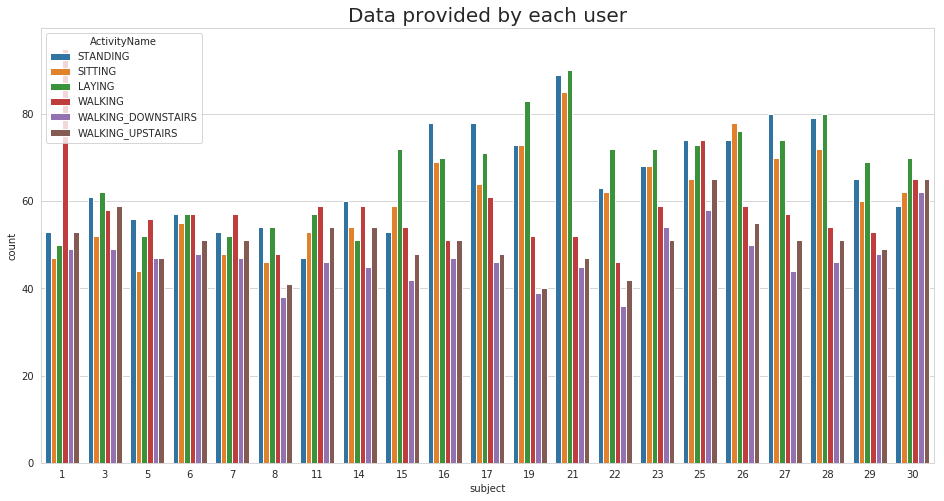We have got almost same number of reading from all the subjects

In :
plt.title('No of Datapoints per Activity', fontsize=15)
sns.countplot(train.ActivityName)
plt.xticks(rotation=90)
plt.show()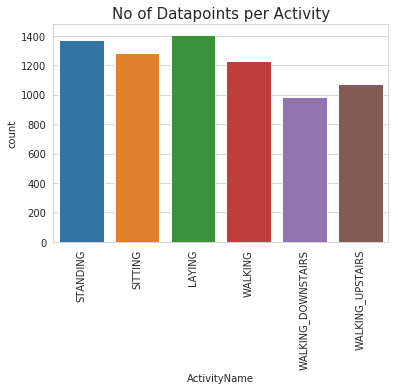### Observation¶

Our data is well balanced (almost)

## 4. Changing feature names¶

In :
columns = train.columns

# Removing '()' from column names
columns = columns.str.replace('[()]','')
columns = columns.str.replace('[-]', '')
columns = columns.str.replace('[,]','')

train.columns = columns
test.columns = columns

test.columns

Out:
Index(['tBodyAccmeanX', 'tBodyAccmeanY', 'tBodyAccmeanZ', 'tBodyAccstdX',
...
'angletBodyAccMeangravity', 'angletBodyAccJerkMeangravityMean',
'angletBodyGyroMeangravityMean', 'angletBodyGyroJerkMeangravityMean',
'angleXgravityMean', 'angleYgravityMean', 'angleZgravityMean',
'subject', 'Activity', 'ActivityName'],
dtype='object', length=564)

## 5. Save this dataframe in a csv files¶

In :
train.to_csv('drive/My Drive/Applied_AI/HAR/UCI_HAR_Dataset/csv_files/train.csv', index=False)
test.to_csv('drive/My Drive/Applied_AI/HAR/UCI_HAR_Dataset/csv_files/test.csv', index=False)


# Exploratory Data Analysis¶

"Without domain knowledge EDA has no meaning, without EDA a problem has no soul."

### 1. Featuring Engineering from Domain Knowledge¶

• Static and Dynamic Activities

• In static activities (sit, stand, lie down) motion information will not be very useful.
• In the dynamic activities (Walking, WalkingUpstairs,WalkingDownstairs) motion info will be significant.

### 2. Stationary and Moving activities are completely different¶

In :
sns.set_palette("Set1", desat=0.80)
facetgrid = sns.FacetGrid(train, hue='ActivityName', size=6,aspect=2)
facetgrid.map(sns.distplot,'tBodyAccMagmean', hist=False)\
plt.annotate("Stationary Activities", xy=(-0.956,17), xytext=(-0.9, 23), size=20,\
va='center', ha='left',\

plt.annotate("Moving Activities", xy=(0,3), xytext=(0.2, 9), size=20,\
va='center', ha='left',\
plt.show()

/usr/local/lib/python3.6/dist-packages/seaborn/axisgrid.py:230: UserWarning: The size paramter has been renamed to height; please update your code.
warnings.warn(msg, UserWarning)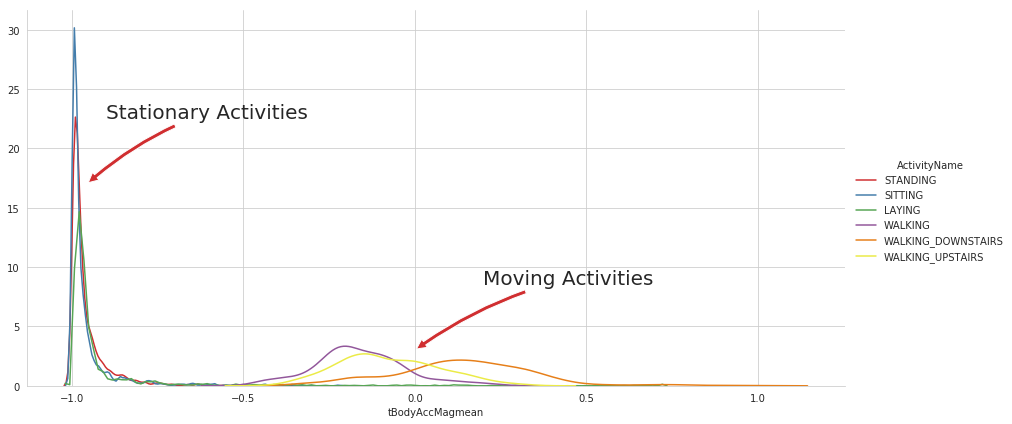In :
# for plotting purposes taking datapoints of each activity to a different dataframe
df1 = train[train['Activity']==1]
df2 = train[train['Activity']==2]
df3 = train[train['Activity']==3]
df4 = train[train['Activity']==4]
df5 = train[train['Activity']==5]
df6 = train[train['Activity']==6]

plt.figure(figsize=(14,7))
plt.subplot(2,2,1)
plt.title('Stationary Activities(Zoomed in)')
sns.distplot(df4['tBodyAccMagmean'],color = 'r',hist = False, label = 'Sitting')
sns.distplot(df5['tBodyAccMagmean'],color = 'm',hist = False,label = 'Standing')
sns.distplot(df6['tBodyAccMagmean'],color = 'c',hist = False, label = 'Laying')
plt.axis([-1.01, -0.5, 0, 35])
plt.legend(loc='center')

plt.subplot(2,2,2)
plt.title('Moving Activities')
sns.distplot(df1['tBodyAccMagmean'],color = 'red',hist = False, label = 'Walking')
sns.distplot(df2['tBodyAccMagmean'],color = 'blue',hist = False,label = 'Walking Up')
sns.distplot(df3['tBodyAccMagmean'],color = 'green',hist = False, label = 'Walking down')
plt.legend(loc='center right')

plt.tight_layout()
plt.show()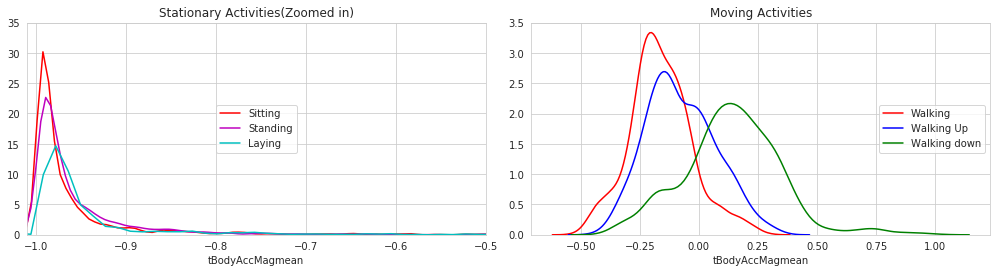### 3. Magnitude of an acceleration can saperate it well¶

In :
plt.figure(figsize=(7,7))
sns.boxplot(x='ActivityName', y='tBodyAccMagmean',data=train, showfliers=False, saturation=1)
plt.ylabel('Acceleration Magnitude mean')
plt.axhline(y=-0.7, xmin=0.1, xmax=0.9,dashes=(5,5), c='g')
plt.axhline(y=-0.05, xmin=0.4, dashes=(5,5), c='m')
plt.xticks(rotation=90)
plt.show()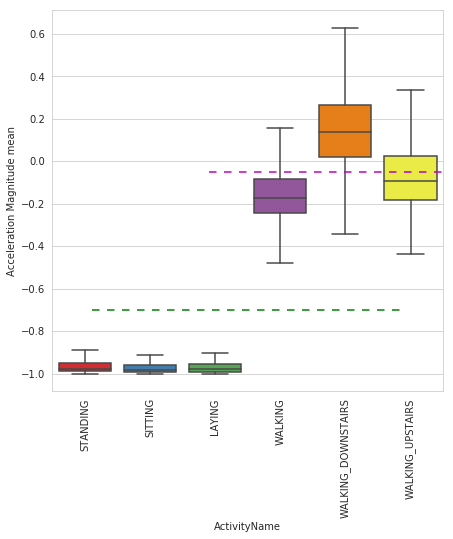Observations:

• If tAccMean is < -0.8 then the Activities are either Standing or Sitting or Laying.
• If tAccMean is > -0.6 then the Activities are either Walking or WalkingDownstairs or WalkingUpstairs.
• If tAccMean > 0.0 then the Activity is WalkingDownstairs.
• We can classify 75% the Acitivity labels with some errors.

### 4. Position of GravityAccelerationComponants also matters¶

In :
sns.boxplot(x='ActivityName', y='angleXgravityMean', data=train)
plt.axhline(y=0.08, xmin=0.1, xmax=0.9,c='m',dashes=(5,3))
plt.title('Angle between X-axis and Gravity_mean', fontsize=15)
plt.xticks(rotation = 40)
plt.show()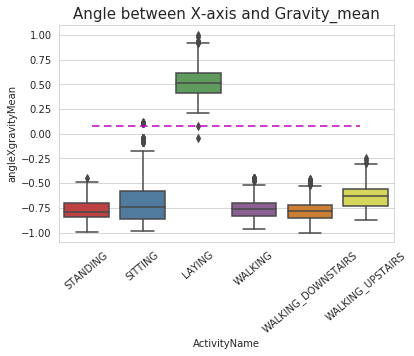Observations:

• If angleX,gravityMean > 0 then Activity is Laying.
• We can classify all datapoints belonging to Laying activity with just a single if else statement.
In :
sns.boxplot(x='ActivityName', y='angleYgravityMean', data = train, showfliers=False)
plt.title('Angle between Y-axis and Gravity_mean', fontsize=15)
plt.xticks(rotation = 40)
plt.axhline(y=-0.22, xmin=0.1, xmax=0.8, dashes=(5,3), c='m')
plt.show()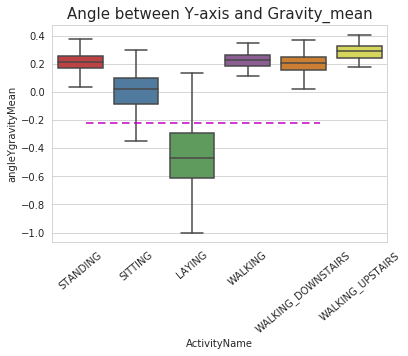# Apply t-sne on the data¶

In :
import numpy as np
from sklearn.manifold import TSNE
import matplotlib.pyplot as plt
import seaborn as sns

In :
# performs t-sne with different perplexity values and their repective plots..

def perform_tsne(X_data, y_data, perplexities, n_iter=1000, img_name_prefix='t-sne'):

for index,perplexity in enumerate(perplexities):
# perform t-sne
print('\nperforming tsne with perplexity {} and with {} iterations at max'.format(perplexity, n_iter))
X_reduced = TSNE(verbose=2, perplexity=perplexity).fit_transform(X_data)
print('Done..')

# prepare the data for seaborn
print('Creating plot for this t-sne visualization..')
df = pd.DataFrame({'x':X_reduced[:,0], 'y':X_reduced[:,1] ,'label':y_data})

# draw the plot in appropriate place in the grid
sns.lmplot(data=df, x='x', y='y', hue='label', fit_reg=False, size=8,\
palette="Set1",markers=['^','v','s','o', '1','2'])
plt.title("perplexity : {} and max_iter : {}".format(perplexity, n_iter))
img_name = img_name_prefix + '_perp_{}_iter_{}.png'.format(perplexity, n_iter)
print('saving this plot as image in present working directory...')
plt.savefig(img_name)
plt.show()
print('Done')

In :
X_pre_tsne = train.drop(['subject', 'Activity','ActivityName'], axis=1)
y_pre_tsne = train['ActivityName']
perform_tsne(X_data = X_pre_tsne,y_data=y_pre_tsne, perplexities =[2,5,10,20,50])

performing tsne with perplexity 2 and with 1000 iterations at max
[t-SNE] Computing 7 nearest neighbors...
[t-SNE] Indexed 7352 samples in 0.426s...
[t-SNE] Computed neighbors for 7352 samples in 72.001s...
[t-SNE] Computed conditional probabilities for sample 1000 / 7352
[t-SNE] Computed conditional probabilities for sample 2000 / 7352
[t-SNE] Computed conditional probabilities for sample 3000 / 7352
[t-SNE] Computed conditional probabilities for sample 4000 / 7352
[t-SNE] Computed conditional probabilities for sample 5000 / 7352
[t-SNE] Computed conditional probabilities for sample 6000 / 7352
[t-SNE] Computed conditional probabilities for sample 7000 / 7352
[t-SNE] Computed conditional probabilities for sample 7352 / 7352
[t-SNE] Mean sigma: 0.635855
[t-SNE] Computed conditional probabilities in 0.071s
[t-SNE] Iteration 50: error = 124.8017578, gradient norm = 0.0253939 (50 iterations in 16.625s)
[t-SNE] Iteration 100: error = 107.2019501, gradient norm = 0.0284782 (50 iterations in 9.735s)
[t-SNE] Iteration 150: error = 100.9872894, gradient norm = 0.0185151 (50 iterations in 5.346s)
[t-SNE] Iteration 200: error = 97.6054382, gradient norm = 0.0142084 (50 iterations in 7.013s)
[t-SNE] Iteration 250: error = 95.3084183, gradient norm = 0.0132592 (50 iterations in 5.703s)
[t-SNE] KL divergence after 250 iterations with early exaggeration: 95.308418
[t-SNE] Iteration 300: error = 4.1209540, gradient norm = 0.0015668 (50 iterations in 7.156s)
[t-SNE] Iteration 350: error = 3.2113254, gradient norm = 0.0009953 (50 iterations in 8.022s)
[t-SNE] Iteration 400: error = 2.7819963, gradient norm = 0.0007203 (50 iterations in 9.419s)
[t-SNE] Iteration 450: error = 2.5178111, gradient norm = 0.0005655 (50 iterations in 9.370s)
[t-SNE] Iteration 500: error = 2.3341548, gradient norm = 0.0004804 (50 iterations in 7.681s)
[t-SNE] Iteration 550: error = 2.1961622, gradient norm = 0.0004183 (50 iterations in 7.097s)
[t-SNE] Iteration 600: error = 2.0867445, gradient norm = 0.0003664 (50 iterations in 9.274s)
[t-SNE] Iteration 650: error = 1.9967778, gradient norm = 0.0003279 (50 iterations in 7.697s)
[t-SNE] Iteration 700: error = 1.9210005, gradient norm = 0.0002984 (50 iterations in 8.174s)
[t-SNE] Iteration 750: error = 1.8558111, gradient norm = 0.0002776 (50 iterations in 9.747s)
[t-SNE] Iteration 800: error = 1.7989457, gradient norm = 0.0002569 (50 iterations in 8.687s)
[t-SNE] Iteration 850: error = 1.7490212, gradient norm = 0.0002394 (50 iterations in 8.407s)
[t-SNE] Iteration 900: error = 1.7043383, gradient norm = 0.0002224 (50 iterations in 8.351s)
[t-SNE] Iteration 950: error = 1.6641431, gradient norm = 0.0002098 (50 iterations in 7.841s)
[t-SNE] Iteration 1000: error = 1.6279151, gradient norm = 0.0001989 (50 iterations in 5.623s)
[t-SNE] Error after 1000 iterations: 1.627915
Done..
Creating plot for this t-sne visualization..
saving this plot as image in present working directory...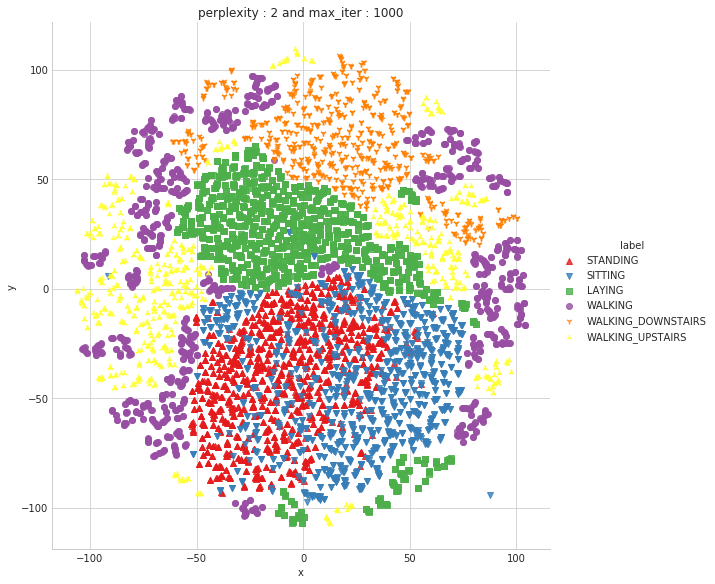Done

performing tsne with perplexity 5 and with 1000 iterations at max
[t-SNE] Computing 16 nearest neighbors...
[t-SNE] Indexed 7352 samples in 0.263s...
[t-SNE] Computed neighbors for 7352 samples in 48.983s...
[t-SNE] Computed conditional probabilities for sample 1000 / 7352
[t-SNE] Computed conditional probabilities for sample 2000 / 7352
[t-SNE] Computed conditional probabilities for sample 3000 / 7352
[t-SNE] Computed conditional probabilities for sample 4000 / 7352
[t-SNE] Computed conditional probabilities for sample 5000 / 7352
[t-SNE] Computed conditional probabilities for sample 6000 / 7352
[t-SNE] Computed conditional probabilities for sample 7000 / 7352
[t-SNE] Computed conditional probabilities for sample 7352 / 7352
[t-SNE] Mean sigma: 0.961265
[t-SNE] Computed conditional probabilities in 0.122s
[t-SNE] Iteration 50: error = 114.1862640, gradient norm = 0.0184120 (50 iterations in 55.655s)
[t-SNE] Iteration 100: error = 97.6535568, gradient norm = 0.0174309 (50 iterations in 12.580s)
[t-SNE] Iteration 150: error = 93.1900101, gradient norm = 0.0101048 (50 iterations in 9.180s)
[t-SNE] Iteration 200: error = 91.2315445, gradient norm = 0.0074560 (50 iterations in 10.340s)
[t-SNE] Iteration 250: error = 90.0714417, gradient norm = 0.0057667 (50 iterations in 9.458s)
[t-SNE] KL divergence after 250 iterations with early exaggeration: 90.071442
[t-SNE] Iteration 300: error = 3.5796804, gradient norm = 0.0014691 (50 iterations in 8.718s)
[t-SNE] Iteration 350: error = 2.8173938, gradient norm = 0.0007508 (50 iterations in 10.180s)
[t-SNE] Iteration 400: error = 2.4344938, gradient norm = 0.0005251 (50 iterations in 10.506s)
[t-SNE] Iteration 450: error = 2.2156141, gradient norm = 0.0004069 (50 iterations in 10.072s)
[t-SNE] Iteration 500: error = 2.0703306, gradient norm = 0.0003340 (50 iterations in 10.511s)
[t-SNE] Iteration 550: error = 1.9646366, gradient norm = 0.0002816 (50 iterations in 9.792s)
[t-SNE] Iteration 600: error = 1.8835558, gradient norm = 0.0002471 (50 iterations in 9.098s)
[t-SNE] Iteration 650: error = 1.8184001, gradient norm = 0.0002184 (50 iterations in 8.656s)
[t-SNE] Iteration 700: error = 1.7647167, gradient norm = 0.0001961 (50 iterations in 9.063s)
[t-SNE] Iteration 750: error = 1.7193680, gradient norm = 0.0001796 (50 iterations in 9.754s)
[t-SNE] Iteration 800: error = 1.6803776, gradient norm = 0.0001655 (50 iterations in 9.540s)
[t-SNE] Iteration 850: error = 1.6465144, gradient norm = 0.0001538 (50 iterations in 9.953s)
[t-SNE] Iteration 900: error = 1.6166563, gradient norm = 0.0001421 (50 iterations in 10.270s)
[t-SNE] Iteration 950: error = 1.5901035, gradient norm = 0.0001335 (50 iterations in 6.609s)
[t-SNE] Iteration 1000: error = 1.5664237, gradient norm = 0.0001257 (50 iterations in 8.553s)
[t-SNE] Error after 1000 iterations: 1.566424
Done..
Creating plot for this t-sne visualization..
saving this plot as image in present working directory...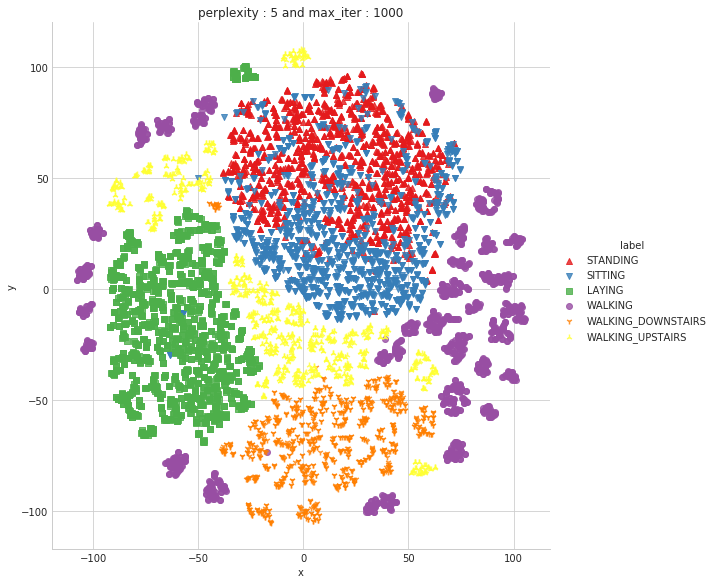Done

performing tsne with perplexity 10 and with 1000 iterations at max
[t-SNE] Computing 31 nearest neighbors...
[t-SNE] Indexed 7352 samples in 0.410s...
[t-SNE] Computed neighbors for 7352 samples in 64.801s...
[t-SNE] Computed conditional probabilities for sample 1000 / 7352
[t-SNE] Computed conditional probabilities for sample 2000 / 7352
[t-SNE] Computed conditional probabilities for sample 3000 / 7352
[t-SNE] Computed conditional probabilities for sample 4000 / 7352
[t-SNE] Computed conditional probabilities for sample 5000 / 7352
[t-SNE] Computed conditional probabilities for sample 6000 / 7352
[t-SNE] Computed conditional probabilities for sample 7000 / 7352
[t-SNE] Computed conditional probabilities for sample 7352 / 7352
[t-SNE] Mean sigma: 1.133828
[t-SNE] Computed conditional probabilities in 0.214s
[t-SNE] Iteration 50: error = 106.0169220, gradient norm = 0.0194293 (50 iterations in 24.550s)
[t-SNE] Iteration 100: error = 90.3036194, gradient norm = 0.0097653 (50 iterations in 11.936s)
[t-SNE] Iteration 150: error = 87.3132935, gradient norm = 0.0053059 (50 iterations in 11.246s)
[t-SNE] Iteration 200: error = 86.1169128, gradient norm = 0.0035844 (50 iterations in 11.864s)
[t-SNE] Iteration 250: error = 85.4133606, gradient norm = 0.0029100 (50 iterations in 11.944s)
[t-SNE] KL divergence after 250 iterations with early exaggeration: 85.413361
[t-SNE] Iteration 300: error = 3.1394315, gradient norm = 0.0013976 (50 iterations in 11.742s)
[t-SNE] Iteration 350: error = 2.4929206, gradient norm = 0.0006466 (50 iterations in 11.627s)
[t-SNE] Iteration 400: error = 2.1733041, gradient norm = 0.0004230 (50 iterations in 11.846s)
[t-SNE] Iteration 450: error = 1.9884514, gradient norm = 0.0003124 (50 iterations in 11.405s)
[t-SNE] Iteration 500: error = 1.8702440, gradient norm = 0.0002514 (50 iterations in 11.320s)
[t-SNE] Iteration 550: error = 1.7870129, gradient norm = 0.0002107 (50 iterations in 12.009s)
[t-SNE] Iteration 600: error = 1.7246909, gradient norm = 0.0001824 (50 iterations in 10.632s)
[t-SNE] Iteration 650: error = 1.6758548, gradient norm = 0.0001590 (50 iterations in 11.270s)
[t-SNE] Iteration 700: error = 1.6361949, gradient norm = 0.0001451 (50 iterations in 12.072s)
[t-SNE] Iteration 750: error = 1.6034756, gradient norm = 0.0001305 (50 iterations in 11.607s)
[t-SNE] Iteration 800: error = 1.5761518, gradient norm = 0.0001188 (50 iterations in 9.409s)
[t-SNE] Iteration 850: error = 1.5527289, gradient norm = 0.0001113 (50 iterations in 8.309s)
[t-SNE] Iteration 900: error = 1.5328671, gradient norm = 0.0001021 (50 iterations in 9.433s)
[t-SNE] Iteration 950: error = 1.5152045, gradient norm = 0.0000974 (50 iterations in 11.488s)
[t-SNE] Iteration 1000: error = 1.4999681, gradient norm = 0.0000933 (50 iterations in 10.593s)
[t-SNE] Error after 1000 iterations: 1.499968
Done..
Creating plot for this t-sne visualization..
saving this plot as image in present working directory...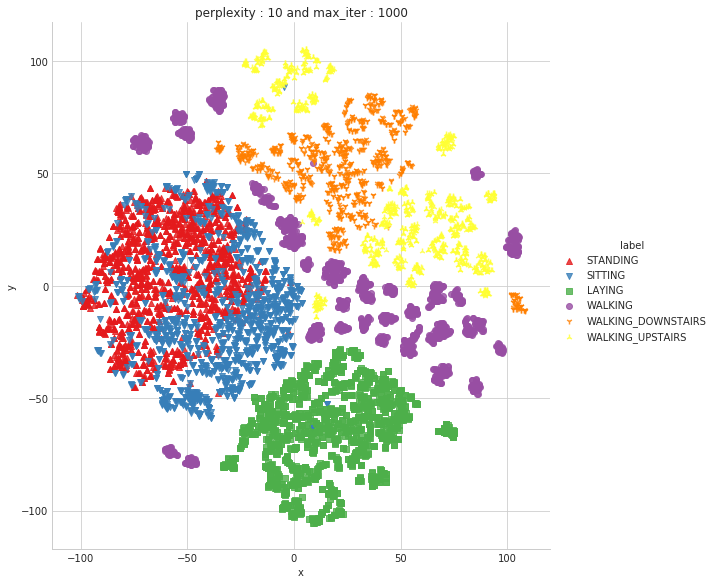Done

performing tsne with perplexity 20 and with 1000 iterations at max
[t-SNE] Computing 61 nearest neighbors...
[t-SNE] Indexed 7352 samples in 0.425s...
[t-SNE] Computed neighbors for 7352 samples in 61.792s...
[t-SNE] Computed conditional probabilities for sample 1000 / 7352
[t-SNE] Computed conditional probabilities for sample 2000 / 7352
[t-SNE] Computed conditional probabilities for sample 3000 / 7352
[t-SNE] Computed conditional probabilities for sample 4000 / 7352
[t-SNE] Computed conditional probabilities for sample 5000 / 7352
[t-SNE] Computed conditional probabilities for sample 6000 / 7352
[t-SNE] Computed conditional probabilities for sample 7000 / 7352
[t-SNE] Computed conditional probabilities for sample 7352 / 7352
[t-SNE] Mean sigma: 1.274335
[t-SNE] Computed conditional probabilities in 0.355s
[t-SNE] Iteration 50: error = 97.5202179, gradient norm = 0.0223863 (50 iterations in 21.168s)
[t-SNE] Iteration 100: error = 83.9500732, gradient norm = 0.0059110 (50 iterations in 17.306s)
[t-SNE] Iteration 150: error = 81.8804779, gradient norm = 0.0035797 (50 iterations in 14.258s)
[t-SNE] Iteration 200: error = 81.1615143, gradient norm = 0.0022536 (50 iterations in 14.130s)
[t-SNE] Iteration 250: error = 80.7704086, gradient norm = 0.0018108 (50 iterations in 15.340s)
[t-SNE] KL divergence after 250 iterations with early exaggeration: 80.770409
[t-SNE] Iteration 300: error = 2.6957574, gradient norm = 0.0012993 (50 iterations in 13.605s)
[t-SNE] Iteration 350: error = 2.1637220, gradient norm = 0.0005765 (50 iterations in 13.248s)
[t-SNE] Iteration 400: error = 1.9143614, gradient norm = 0.0003474 (50 iterations in 14.774s)
[t-SNE] Iteration 450: error = 1.7684202, gradient norm = 0.0002458 (50 iterations in 15.502s)
[t-SNE] Iteration 500: error = 1.6744757, gradient norm = 0.0001923 (50 iterations in 14.808s)
[t-SNE] Iteration 550: error = 1.6101606, gradient norm = 0.0001575 (50 iterations in 14.043s)
[t-SNE] Iteration 600: error = 1.5641028, gradient norm = 0.0001344 (50 iterations in 15.769s)
[t-SNE] Iteration 650: error = 1.5291905, gradient norm = 0.0001182 (50 iterations in 15.834s)
[t-SNE] Iteration 700: error = 1.5024391, gradient norm = 0.0001055 (50 iterations in 15.398s)
[t-SNE] Iteration 750: error = 1.4809053, gradient norm = 0.0000965 (50 iterations in 14.594s)
[t-SNE] Iteration 800: error = 1.4631859, gradient norm = 0.0000884 (50 iterations in 15.025s)
[t-SNE] Iteration 850: error = 1.4486470, gradient norm = 0.0000832 (50 iterations in 14.060s)
[t-SNE] Iteration 900: error = 1.4367288, gradient norm = 0.0000804 (50 iterations in 12.389s)
[t-SNE] Iteration 950: error = 1.4270191, gradient norm = 0.0000761 (50 iterations in 10.392s)
[t-SNE] Iteration 1000: error = 1.4189968, gradient norm = 0.0000787 (50 iterations in 12.355s)
[t-SNE] Error after 1000 iterations: 1.418997
Done..
Creating plot for this t-sne visualization..
saving this plot as image in present working directory...
`